# There 13

There are 7 cows 3 sheeps and 4 chickens on a field . What fraction of the animals are sheep?

f =  0.2143

### Step-by-step explanation: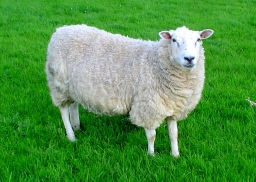Did you find an error or inaccuracy? Feel free to write us. Thank you!Tips to related online calculators
Need help to calculate sum, simplify or multiply fractions? Try our fraction calculator.

## Related math problems and questions:

• Sheep and cowsThere are only sheep and cows on the farm. Sheep is eight more than cows. The number of cows is half the number of sheep. How many animals live on the farm?On the meadow grazing horses, cows, and sheep, together with less than 200. If cows were 45 times more, horses 60 times more, and sheep 35 times more than there are now, their numbers would equally. How many horses, cows, and sheep are on the meadow toget
• Fewer than 500 sheep,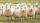There are fewer than 500 sheep, but if they stand in a double, triple, quadruple, five, and sixth-order, one sheep will remain. But they can stand in the seventh order. How many are sheep?
• Animal species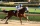Of 100 types of animals, 9/100 were discovered in ancient times, and 2/100 were discovered in the Middle Ages. Another 3/10 were discovered in the 1800s. What fraction of the 100 types of animals was discovered after the 1800s? Explain.
• Shepherd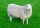Kuba makes a deal with a shepherd to take care of his sheep. Shepherd said to Kuba that he would receive twenty gold coins and one sheep after a year of service. But Kuba resigned just after the seventh month of service. But shepherd rewarded him and paid
• Animals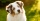All without two animals are dogs, all without two are parrots, all without two are cats, the others are chickens. How many animals of individual species does Ms. Novak take care of?
• The farmer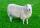The farmer had 140 sheep. For the next year, she decided to change the number of sheep in ratio 10: 7. How many sheep will he have then?
• FractionFind for what x fraction (-4x -6)/(x) equals:
• Cows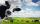4 cows spent 16 bags of hay in 5 days. How many bags of hay sacks are needed for 5 cows for seven days?
• Cows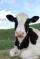20 cows has food for 60 days. We reduce the dose to two-thirds for 18 cows. How many days will be fed?
• What fraction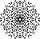What fraction of number 1 to 30 is prime?
• Division by zero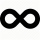Fraction 5 by 2. if 3 is added to numerator and 2 is subtracted from the denominator then the new fraction is:
• Equation with fractionsWhat is the simplified form of the equation fraction 4 over 5 n minus fraction 1 over 5 equals fraction 2 over 5 n? n = -2 n = 4 n = fraction 1 over 2
• CompareCompare fractions (34)/(3) and (12)/(4). Which fraction of the lower?
• Simplest form of a fractionWhich one of the following fraction after reducing in simplest form is not equal to 3/2? a) 15/20 b) 12/8 c) 27/18 d) 6/4
• A fractionI think of a fraction, if we increase its numerator and denominator by one, the value of the fraction increases by one tenth, what fraction do I think?
• Shepherd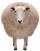The shepherd has fewer than 500 sheep; where they can be up to 2, 3, 4, 5, 6 row is always 1 remain, and as can be increased up to 7 rows of the sheep, and it is not increased no ovine. How many sheep has a shepherd?